# Nintenren Free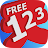100+Everyone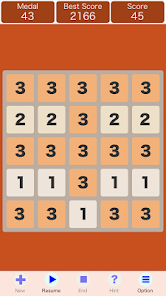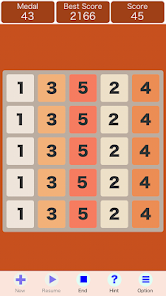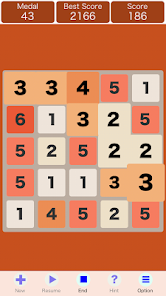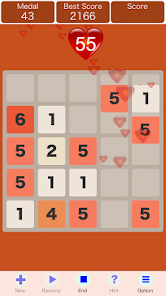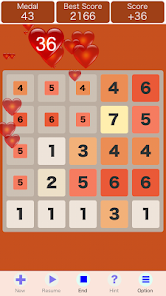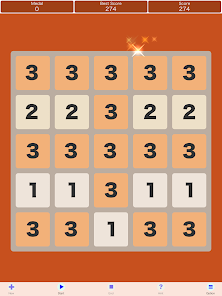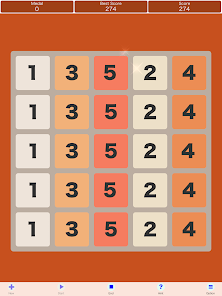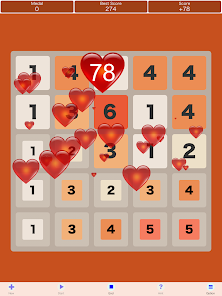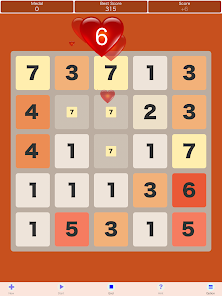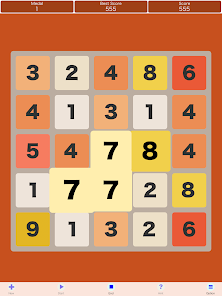This is a casual and relaxing game. Your goal is to join numbers together and remove them to get scores as high as possible until the game comes to the end.

◎How to join numbers together
Two numbers can be joined together if they are of the same value, or difference of 1.
(e.g. 11, 23, 32)

◎How to remove numbers
You must join at least 3 different numbers or 3 same numbers together.
(e.g. 123, 222, 5433)

◎How to calculate scores
The score of each number equals to its order.
(e.g. Removing 3 numbers gets 6 points (1+2+3=6); removing 4 numbers gets 10 points (1+2+3+4=10); removing 5 numbers gets 15 points (1+2+3+4+5=15).

◎How to get high score
The longer the chain of the numbers is, the higher the score is. On the other hand, pursuing long numbers without strategy will probably end the game.
Updated on
Aug 1, 2018

## Data safety